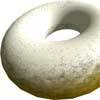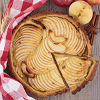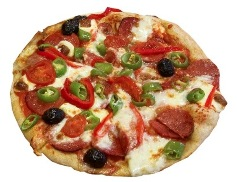#### You may also like### Doughnut

How can you cut a doughnut into 8 equal pieces with only three cuts of a knife?### Pies

Grandma found her pie balanced on the scale with two weights and a quarter of a pie. So how heavy was each pie?### Red Balloons, Blue Balloons

Katie and Will have some balloons. Will's balloon burst at exactly the same size as Katie's at the beginning of a puff. How many puffs had Will done before his balloon burst?

# Pizza Portions

##### Age 7 to 11Challenge Level

My friends and I love pizza.This is great because we always know what kind of food to make. There is a small problem. We often end up arguing over how much each person has eaten. We like to be fair and share out the pizzas equally.

Can you help us?

If I had $1$ pizza and wanted to share it equally between $2$ of us, how much pizza would we get each?

How much would each of us have if we had $1$ pizza to share equally between 3 people?

Sometimes, we have some pizza left over which we reheat the next day.

If I have $1/2$ a pizza and I want to split it between $2$ of us, what fraction of the whole pizza do we each get?

If there were $1/3$ of a pizza left and $2$ of us to share it, what fraction of the whole pizza would we get now?
How about if there were $3$ of us and we had $1/4$ of a pizza to share?

Look back at what you've done so far. Can you write down how you worked out your answers using numbers and maths symbols?

Can you see any patterns in the numbers that you've used?

Today, each of us would like $1/2$ a pizza. If I have $1$ pizza, how many people can have $1/2$ a pizza?

If I had $2$ pizzas altogether how many people can have $1/2$ a pizza each now?

Can you think of a way to write this using numbers and symbols?

Can you see any patterns in the numbers this time?

Sometimes, we may be a little hungrier so we'd like $2/3$ of a pizza each.

How many pizzas would I need to buy for $3$ people?

How many for $4$ of us? Would there be any left over?

How could you write this using numbers and symbols?